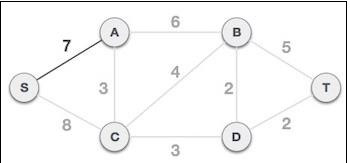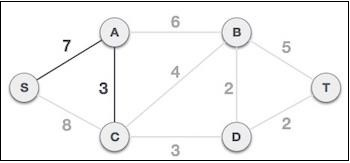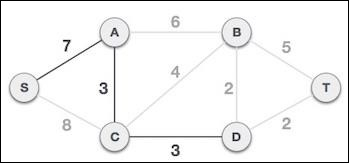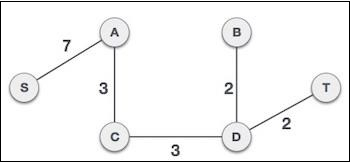# Prim's algorithm in Javascript

Prim's algorithm is a greedy algorithm that finds a minimum spanning tree for a weighted undirected graph. It finds a subset of the edges that forms a tree that includes every vertex, where the total weight of all the edges in the tree is minimized.

The algorithm operates by building this tree one vertex at a time, from an arbitrary starting vertex, at each step adding the cheapest possible connection from the tree to another vertex.

## How does Prim's algorithm work?

Let us look at an illustration of how Prim's algorithm works −

1. Choose any arbitrary node as the root node: In this case, we choose S node as the root node of Prim's spanning tree. This node is arbitrarily chosen, so any node can be the root node. One may wonder why any video can be a root node. So the answer is, in the spanning tree all the nodes of a graph are included and because it is connected then there must be at least one edge, which will join it to the rest of the tree.

2. Check outgoing edges and select the one with less cost: After choosing the root node S, we see that S, A, and S, C are two edges with weight 7 and 8, respectively. We choose the edge S, A as it is lesser than the other.Now, the tree S-7-A is treated as one node and we check for all edges going out from it. We select the one which has the lowest cost and include it in the tree.After this step, the S-7-A-3-C tree is formed. Now we'll again treat it as a node and will check all the edges again. However, we will choose only the least cost edge. In this case, C-3-D is the new edge, which is less than other edges' cost 8, 6, 4, etc.After adding node D to the spanning tree, we now have two edges going out of it having the same cost, i.e. D-2-T and D-2-B. Thus, we can add either one. But the next step will again yield edge 2 as the least cost. Hence, we are showing a spanning tree with both edges included.Now let us see how we can implement the same in our code −

## Example

primsMST() {
// Initialize graph that'll contain the MST
const MST = new Graph();
if (this.nodes.length === 0) {
return MST;
}

// Select first node as starting node
let s = this.nodes;

// Create a Priority Queue and explored set
let edgeQueue = new PriorityQueue(this.nodes.length * this.nodes.length);
let explored = new Set();

// Add all edges from this starting node to the PQ taking weights as priority
this.edges[s].forEach(edge => {
edgeQueue.enqueue([s, edge.node], edge.weight);
});

// Take the smallest edge and add that to the new graph
let currentMinEdge = edgeQueue.dequeue();
while (!edgeQueue.isEmpty()) {

// COntinue removing edges till we get an edge with an unexplored node
while (!edgeQueue.isEmpty() && explored.has(currentMinEdge.data)) {
currentMinEdge = edgeQueue.dequeue();
}
let nextNode = currentMinEdge.data;

// Check again as queue might get empty without giving back unexplored element
if (!explored.has(nextNode)) {
// Again add all edges to the PQ
this.edges[nextNode].forEach(edge => {
edgeQueue.enqueue([nextNode, edge.node], edge.weight);
});

// Mark this node as explored explored.add(nextNode);
s = nextNode;
}
}
return MST;
}

You can test this using:

let g = new Graph();

g.primsMST().display();

## Output

This will give the output −

A->B, D
B->A, G
D->A, C, E
C->D
E->D, F
G->B
F->E

Note that our Initial graph was −

## Example

/**
*         A
*       / | \
*       C | B
*       \ | |
*         D G
*         | /
*         E
*         |
*         F
*/

## Output

Our current graph looks like −

/**
*         A
*         |\
*     C   | B
*      \  | |
*       D   G
*       |
*       E
*       |
*       F
*
*/

We've removed the costliest edges and now have a spanning tree.